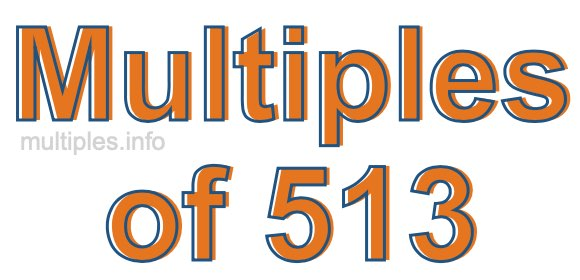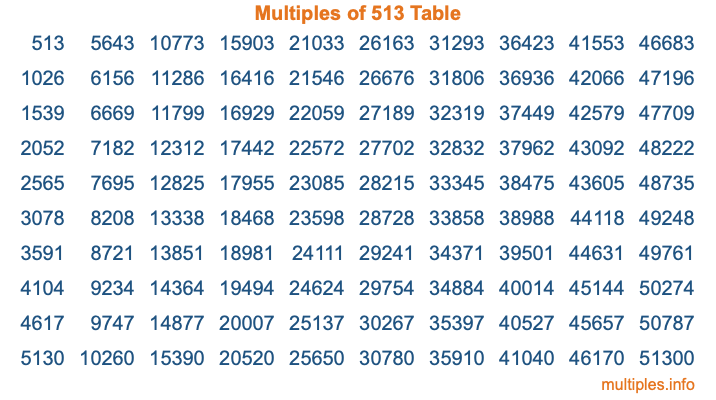Multiples of 513Welcome to the Multiples of 513 page. Here we will first teach you everything you will ever need to know about the multiples of 513, and then give you a study guide summary of everything we taught you to make sure you remember it all. Use this page to look up facts and learn information about the multiples of 513. This page will make you a multiples of five hundred thirteen expert!

Definition of Multiples of 513
Multiples of 513 are all the numbers that when divided by 513 equal an integer. Each of the multiples of 513 are called a multiple. A multiple of 513 is created by multiplying 513 by an integer.

Therefore, to create a list of multiples of 513, you start with 1 multiplied by 513, then 2 multiplied by 513, then 3 multiplied by 513, and so on for as long as you want. Thus, the list of the first five multiples of 513 is 513, 1026, 1539, 2052, and 2565. To see a larger list of multiples of 513, see the printable image of Multiples of 513 further down on this page. We also have a category where you can choose any nth multiple of 513.

Multiples of 513 Checker
The Multiples of 513 Checker below checks to see if any number of your choice is a multiple of 513. In other words, it checks to see if there is any number (integer) that when multiplied by 513 will equal your number. To do that, we divide your number by 513. If the the quotient is an integer, then your number is a multiple of 513.

Is  a multiple of 513?

Least Common Multiple of 513 and ...
A Least Common Multiple (LCM) is the lowest multiple that two or more numbers have in common. This is also called the smallest common multiple or lowest common multiple and is useful to know when you are adding our subtracting fractions. Enter one or more numbers below (513 is already entered) to find the LCM.

Check out our LCM Calculator if you need more details about the Least Common Multiple or if you need the LCM for different numbers for adding and subtraction fractions.

nth Multiple of 513
As we stated above, 513 is the first multiple of 513, 1026 is the second multiple of 513, 1539 is the third multiple of 513, and so on. Enter a number below to find the nth multiple of 513.

th multiple of 513

Multiples of 513 vs Factors of 513
513 is a multiple of 513 and a factor of 513, but that is where the similarities end. All postive multiples of 513 are 513 or greater than 513. All positive factors of 513 are 513 or less than 513.

Below is the beginning list of multiples of 513 and the factors of 513 so you can compare:

Multiples of 513: 513, 1026, 1539, 2052, 2565, etc.

Factors of 513: 1, 3, 9, 19, 27, 57, 171, 513

As you can see, the multiples of 513 are all the numbers that you can divide by 513 to get a whole number. The factors of 513, on the other hand, are all the whole numbers that you can multiply by another whole number to get 513.

It's also interesting to note that if a number (x) is a factor of 513, then 513 will also be a multiple of that number (x).

Multiples of 513 vs Divisors of 513
The divisors of 513 are all the integers that 513 can be divided by evenly. Below is a list of the divisors of 513.

Divisors of 513: 1, 3, 9, 19, 27, 57, 171, 513

The interesting thing to note here is that if you take any multiple of 513 and divide it by a divisor of 513, you will see that the quotient is an integer.

Multiples of 513 Table
Below is an image of the first 100 multiples of 513 in a table. The table is in chronological order, column by column. The first column has the first ten multiples of 513, the second column has the next ten multiples of 513, and so on.The Multiples of 513 Table is also referred to as the 513 Times Table or Times Table of 513. You are welcome to print out our table for your studies.

Negative Multiples of 513
Although not often discussed or needed in math, it is worth mentioning that you can make a list of negative multiples of 513 by multiplying 513 by -1, then by -2, then by -3, and so on, to get the following list of negative multiples of 513:

-513, -1026, -1539, -2052, -2565, etc.

Multiples of 513 Summary
Below is a summary of important Multiples of 513 facts that we have discussed on this page. To retain the knowledge on this page, we recommend that you read through the summary and explain to yourself or a study partner why they hold true.

There are an infinite number of multiples of 513.

A multiple of 513 divided by 513 will equal a whole number.

513 divided by a factor of 513 equals a divisor of 513.

The nth multiple of 513 is n times 513.

The largest factor of 513 is equal to the first positive multiple of 513.

513 is a multiple of every factor of 513.

513 is a multiple of 513.

A multiple of 513 divided by a divisor of 513 equals an integer.

513 divided by a divisor of 513 equals a factor of 513.

Any integer times 513 will equal a multiple of 513.

Multiples of a Number
Here you can get the multiples of another number, all with the same attention to detail as we did for multiples of 513 on this page.

Multiples of
Multiples of 514
Did you find our page about multiples of five hundred thirteen educational? Do you want more knowledge? Check out the multiples of the next number on our list!